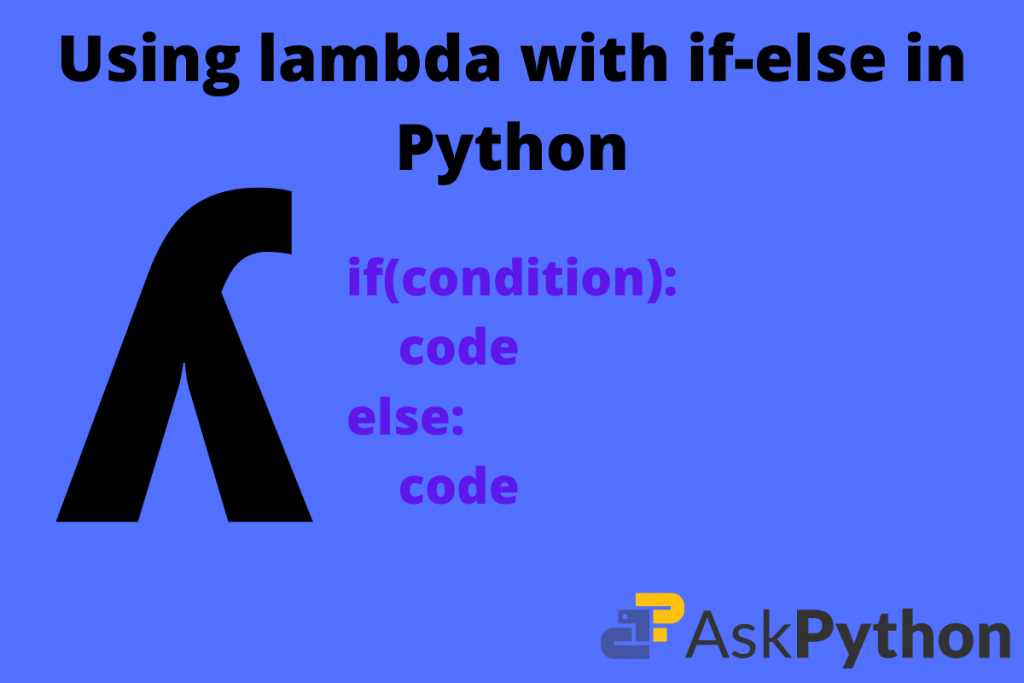# Lambda With Conditional Statements in PythonIn this article, we’ll learn how to use lambda with if-else conditions in Python. Pairing both of these can be very useful when you require more advanced anonymous functions.

Conditional statements in any programming language are one of the fundamental concepts to learn. Their main use is to act according to the conditions provided. Every programmer has to learn these and then proceed towards advanced topics. In almost all languages there are only three conditionals:

1. if
2. else
3. else if

## What is the lambda function in Python?

The lambda function in Python is an anonymous function. The core purpose of using this concept is it makes the creation of functions easy.

### Syntax of Lambda functions

```variable = lambda parameters_list : expression
```

```help('lambda')
```

We get the following output:

```Lambdas
*******

lambda_expr        ::= "lambda" [parameter_list] ":" expression
lambda_expr_nocond ::= "lambda" [parameter_list] ":" expression_nocond

Lambda expressions (sometimes called lambda forms) are used to create
anonymous functions. The expression "lambda parameters: expression"
yields a function object.  The unnamed object behaves like a function
object defined with:

def <lambda>(parameters):
return expression

Note that functions created with lambda expressions cannot contain
statements or annotations.
```

Using lambdas we can carry out the following operations for a beginner-friendly start:

2. Subtraction
3. Multiplication
4. Division

1. Using in functional programming.
2. Use in Object Oriented Programming.
3. Parameterizing a method of a particular class.

Let’s look at a few examples of the lambda function.

Code:

```add = lambda a, b: a + b

# output: 10
```

The code is simple. We first create a lambda object as add. Then we store two parameters inside the lambda expression. These parameters are two numbers that are for the addition operation. After that in the colon, we place the addition expression in front of the colon.

### Subtaction

Code:

```subtract = lambda a, b: a - b

# output: 50
```

### Multiplication

Code:

```multiply = lambda a, b: a * b
print(multiply(100, 50))

# output: 5000
```

## Using Lambda with if-else conditions

Using the lambda with if-else conditions is a useful technique. This also reduces the lines of code. Now, take note that the expression section in lambdas can only hold only one expression at a time.

#### General syntax when using lambda with if-else conditions in Python

```variable_name = lambda parameters : code_for_if if (condition) else code_for_else
```

the syntax has just a bit different from the core implementation of lambda. So, just declare the code before the if statement and then write the if statement with the condition. If the need occurs else block comes directly after the if statement.

```conditional_lambda = lambda x: x/100 if x < 20 else x
print(conditional_lambda(4))

# output: 0.04
```

Explanation:

1. We create a lambda object as conditional_lambda.
2. Then, we store a variable x and expression as x/100 from and in joining with that our conditional statement lies.
3. The statement says that if x is less than 20 divide it by 100 else print it as it is.
4. Then we call the conditional_lambda function and inside it, we place the parameter as 4.
5. As 4 is less than 20 it will get divided by 100 and output is 0.04 on the screen.

Now we shall study how we can use the other advanced operations with this.

Example: Check whether the given number is an even number or odd using lambdas

```# setup lambda
check = lambda num : print(num, 'is Even') if num%2 == 0 else print(num, ' is Odd')

# input from user
num = int(input('Enter any number: '))
a = check(num)
print(a)
```

Output:

```>>> Enter any number: 65
65 is Odd
```
```>>> Enter any number: -56
-56 is Even
```

Example: Check whether the given number is an even number or odd using lambdas

```check = lambda a, b : print(a,'is divisible by', b) if (a%b == 0) else print(a ,' is indivisible by ', b)
a = int(input('Enter value for a: '))
b = int(input('Enter value for b: '))
obj = check(a, b)
```

Output:

```>>> Enter value for a: 45
>>> Enter value for b: 45
45 is divisible by 45
```
```>>> Enter value for a: 3
>>> Enter value for b: 9
3 is indivisible by 9
```

Explanation:

1. First, we define the statement of lambda. The function has two parameters and b. The function name is check.
2. Then after the if block comes. The main condition is a should be divisible by b. If this is true the block prints(“a is divisible by b”). But if the remainder is not zero then the else block prints indivisible.
3. Then we create a function object as obj and call it concerning check(a, b).

### Frame of reference

https://stackoverflow.com/questions/1585322/is-there-a-way-to-perform-if-in-pythons-lambda

## Wrapping up

Thus, we have come to the end of this topic. Using Lambda with if-else conditionals is one of the crucial topics that maintain visibility of one-line codes. The best thing is we do not need to change the core syntax and just edit our conditions and other stuff only. The reader must revise the code and try more than once to understand the code.